Next: Nondilational Chirplet Transform Up: CHIRPLET TRANSFORM SUBSPACES Previous: A Simple Example With

## Relationship Between Auto Chirplet FF Plane and Radon Transform

Conceptually, each point in the FF plane corresponds to a chirp component in the original signal, which also corresponds to a linear portion of the time-frequency (TF) plane. The Radon transform (also known as the Hough transform) is formulated as a family of line integrals through a two-dimensional function. It is known for its ability to extract straight lines from images. For a good survey paper on the Radon transform, see Illingworth and Kittlerillingworth. This property allows us to use it as an alternate means of computing the FF plane of the chirplet transform, by using the TF plane as our input image.

The Radon transform provides us with a simple means of computing the FF plane of the autochirplet transform, by using the Wigner distribution, and arriving at a transform space that tells us basically the same information as the chirplet FF-plane, except that we benefit from the greater resolution of the Wigner distribution. It is well known that the cross components of the Wigner distribution are of an oscillatory nature, while the autocomponents give a net positive contribution. Therefore, since the Radon transform is integrating along lines, the cross terms of the Wigner distribution are cancelled out along each line, so that the points in the Radon transform of the Wigner distribution only ``see'' the autocomponents of the Wigner distribution (Fig. 11(a)).

Figure: FIGURE GOES SOMEWHERE IN THIS GENERAL VICINITY

The Radon transform is usually computed from the normal equation of a line:

x () + y () =

as an integral along each of these lines in the original space. The parameter space is sampled uniformly, in the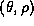coordinates. It is easier to compare the Radon transform of the Wigner distribution with the chirplet CF plane (Fig. 10) if, by first, without loss of generality, we normalize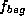and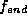to be on the interval from -1/2 to 1/2 and the TF distribution to have time and frequency coordinates on the same interval from -1/2 to 1/2. Then we make the substitution:

() = /f_avg

and

() = 1/f_dif\!f

where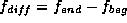and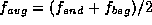.

A simpler (perhaps equally well known) form of the Radon transform parameterizes the lines in terms of their slopes and intercepts. This parameterization has the advantage that it maps lines to points, and points to lines, while it has the disadvantage that there is a singularity when lines of infinite slope (vertical lines) are encountered. Because of the Nyquist limit, however, we do not have this problem when the input to the Radon transform is a time-frequency distribution. Thus we may be tempted to use the slope-intercept form of the Radon transform, except that we would prefer to have a parameterization in that matches the FF plane rather than the CF plane, for reasons previously discussed. The ``Nyquist boundaries'' we referred to earlier are most evident if we simply consider the discrete Radon transform of a matrix of identically non-zero values (Fig. 12), where we can observe the same diamond shape which initially prompted us to useandrather than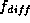and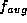.

Figure: FIGURE GOES SOMEWHERE IN THIS GENERAL VICINITY

We may overcome the problems associated with boundaries by defining a new version of the Radon transform, where we use the following pair of parameters:

• Beginning intercept,: the leftmost ordinate on the line (the ordinate for an abscissa of -1/2).
• Ending intercept,: the rightmost ordinate on the line (the ordinate for an abscissa of +1/2).
In Fig. 11(b) we show the autochirplet FF plane calculated from the falling-object data, using the new parameterization of the Radon transform.Next: Nondilational Chirplet Transform Up: CHIRPLET TRANSFORM SUBSPACES Previous: A Simple Example With

Steve Mann
Thu Jan 8 19:50:27 EST 1998